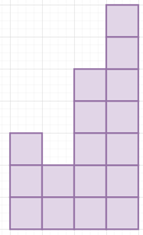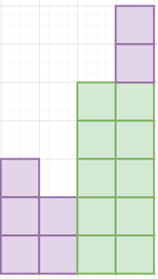# Program to find largest rectangle area under histogram in python

Suppose we have a list of numbers representing heights of bars in a histogram. We have to find area of the largest rectangle that can be formed under the bars.

So, if the input is like nums = [3, 2, 5, 7]then the output will be 10To solve this, we will follow these steps −

• stk := a stack and initially insert -1 into it
• insert 0 at the end of heights
• ans := 0
• for i in range 0 to size of heights, do
• while heights[i] < heights[top of stk], do
• h := heights[top of stk] and pop from stk
• w := i - top of stk - 1
• ans := maximum of ans and (h * w)
• push i into stk
• return ans

Let us see the following implementation to get better understanding −

## Example

Live Demo

class Solution:
def solve(self, heights):
stk = [-1]
heights.append(0)
ans = 0
for i in range(len(heights)):
while heights[i] < heights[stk[-1]]:
h = heights[stk.pop()]
w = i - stk[-1] - 1
ans = max(ans, h * w)
stk.append(i)
return ans

ob = Solution()
nums = [3, 2, 5, 7]
print(ob.solve(nums))

## Input

[3, 2, 5, 7]

## Output

10